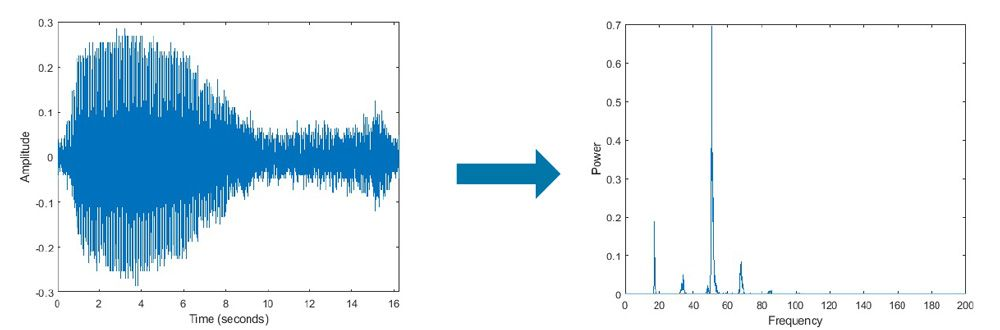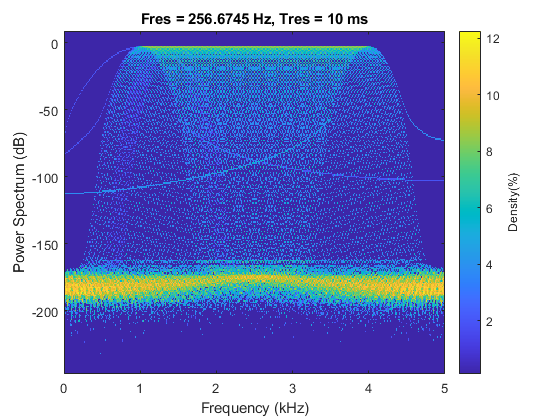# What Is a Fast Fourier Transform (FFT)?

A fast Fourier transform (FFT) is a highly optimized implementation of the discrete Fourier transform (DFT), which convert discrete signals from the time domain to the frequency domain. FFT computations provide information about the frequency content, phase, and other properties of the signal.Blue whale moan audio signal decomposed into its frequency components using FFT. (See MATLAB code example)

Popular FFT algorithms include the Cooley-Tukey algorithm, prime factor FFT algorithm, and Rader’s FFT algorithm. The most commonly used FFT algorithm is the Cooley-Tukey algorithm, which reduces a large DFT into smaller DFTs to increase computation speed and reduce complexity. FFT has applications in many fields.

## FFT Applications

In signal processing, FFT forms the basis of frequency domain analysis (spectral analysis) and is used for signal filtering, spectral estimation, data compression, and other applications. Variations of the FFT such as the short-time Fourier transform also allow for simultaneous analysis in time and frequency domains. These techniques can be used for a variety of signals such as audio and speech, radar, communication, and other sensor data signals. FFT is also sometimes used as an intermediate step for more complex signal processing techniques.

In image processing, FFT is used for filtering and image compression. FFT is also used in physics and mathematics to solve partial differential equations (PDEs).Persistence spectrum, a type of time-frequency view, that can be used for spectral analysis of signals. (See time-frequency functions in MATLAB)

## FFT in MATLAB

MATLAB® provides many functions like fft, ifft, and fft2 with which FFT can be implemented directly. In MATLAB, FFT implementation is optimized to choose from among various FFT algorithms depending on the data size and computation. Similarly, Simulink® provides blocks for FFT that can be used in Model-Based Design and simulation. MATLAB and Simulink also support implementation of FFT on specific hardware such as FPGAs, processors including ARM, and NVIDIA GPUs, through automatic code generation.

Explore the functions and examples below to learn more about Fourier transforms and applications and implementations of FFT using MATLAB.

## Hardware Implementation of FFT

Implementing FFT on programmable logic devices is not as straightforward as software implementation. Incorrect decisions on engineering trade-offs like speed and accuracy or inefficient code can impact the quality and performance of an application. With the MATLAB and Simulink code generation tools, it is easy to implement FFT on various hardware devices, from general-purpose processors such as ARM to more specialized devices such as FPGA.